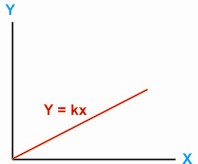HOME MATH DICTIONARY DOWNLOAD FEEDBACK DISCLAIMER
 Question: What is meant by Varies Directly As ? Answer: When two variables such as x and y are related by an equation of the form y = kx. where k is a constant, then y varies directly as x. For example, when a golf ball is dropped from a height x onto a hard surface it will bounce to a height y and where y = kx. This shows on a graph as a straight line through the origin.